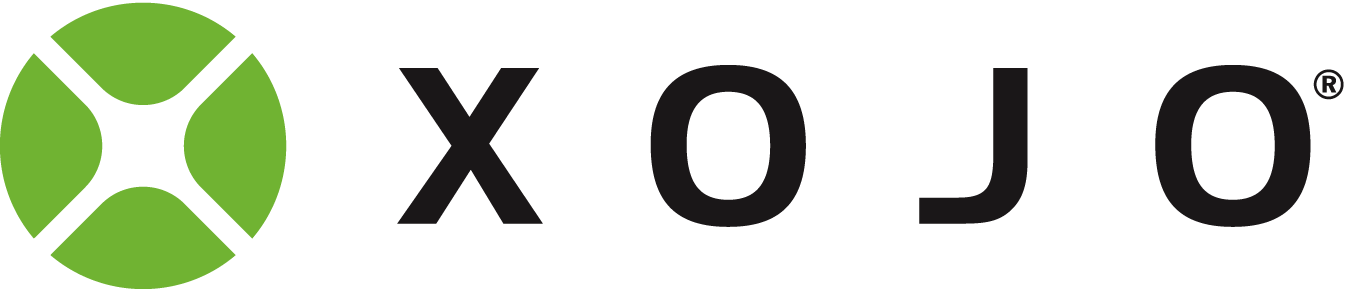# Limiting Value Range

Are there other ways to limit a value in a range of values other then with if/then statements?

If X = range of numbers from 20 to 90 and I wanted Y = X but only from a range of 30 to 70 how might I accomplish that?

Min and Max

``````Value = Max(Min(Value, 70), 30)
``````
2 Likes

That is so clever and exactly what I was looking for. Thanks.

Doesnt this skew the results to give a high incidence of 30 and 70 ?
Happy that this is exactly what you are looking for, but I thought you might want to ‘map’ numbers in the range 20 to 90, to give you a representative range between 30 and 70

A real world example might be that you have a range of values from 30 to 90 and wanted to show this on a dial from 0 to 10 like a volume control, or a percentage meter.

Mapping might go like this:

``````dim srcBase as integer = 20
dim srcMax as integer = 90
dim trgtBase as integer = 30
dim trgtMax as integer = 70
dim newvalue as double
//normalise the value from 0 to ??
newvalue =  value - srcbase  // gets a value between 0 and 70
//compress the value so that it fits in the target 'width'
newvalue = newvalue *  (trgtmax-trgtBase) / (srcMax- srcBase)   'X * 40/70
// push it up into the target range.
newvalue = newvalue + trgtBase
``````

Example :
Consider a value 50, coming from the source range of 20 to 90
Lets see that as a percentage /progress along the source range.

50 is 30 away from the base, and 40 from the top.
A bit under ‘half way’

50-20 = 30
//30 * (trgtmax-trgtBase) / (srcMax- srcBase)
//30 * (100-0) / (90-20)
30 * 100/70 = 42.85
42.85 + 0 = 42.85 %

Thom’s solution code above would have given 50, since 50 is ‘within parameters’
Which is fine
But if anyone wants the values mapped/stretched, the maths above should do it.

1 Like

Maybe I misunderstood, but I think you’re over complicating. If 20 <= X <= 90, Y = Min(Max(X, 30), 70). Basically… the range of X is irrelevant.

1 Like

These ranges are being used to build an algorithm for a heating system. If three parameters are being used, outside temp, inside temp, and outside light condition which determines solar gain, I needed to limit the influence of temperatures. If it’s darn cold out it won’t make much difference if it’s really darn cold out since the boiler will need to be full tilt at darn cold. The other parts of the algorithm would be overwhelmed. Right now I giving weights to the three input sources. 0-15 for inside temp within a range of 60-70 deg, Outside temp, gets 0-10 for a temp range from 20F -70F and outside light gets 0-8 for a full range of 0 to 100% sun. This is a sensor that detects cloud density. The values are additive but limited to a total of 15 which determines the temperature of the water being pumped into the floor system. The mixing valve has an input range of 0-15. Once I’m satisfied with the values I’d like to place a modifier on the outside light input to adjust for sun azimuth according to time of year which affects solar penetration into the home. The home is very passive with a concrete slab radiant floor system hence the need for heat requirement anticipation.

It’s an interesting project so any thoughts on tweaking the algorithm are welcome.1 Like